Question

The radius of curvature of the path of a charged particle in a uniform magnetic field is directly proportional toA) the particle’s charge.B) the particle’s momentum.C) the particle’s energy.D) the flux density of the field.E)All of these are correct

1.B) the particle’s momentum.

Explanation:

We know that

The centripetal force  on the particle when its moving in the radius R and velocity V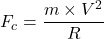The magnetic force on the particle when the its moving with velocity V in the magnetic filed B and having charge q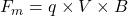At the equilibrium condition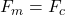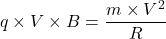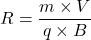Momentum = m V

Therefore we can say that the radius of curvature is directly proportional to the particle momentum.

B) the particle’s momentum.